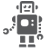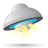## MK64f12-Twr Spi_dma issues

cancel
Showing results for
Did you mean:

## MK64f12-Twr Spi_dma issues

355 ViewsContributor I

Hello everyone

I am trying to implement a simple dma based spi code. I started from the edma_dspi demo code from Kinetis SDK1.0.0 and made some changes to suit my application. I am using a dma channel 0, DMATXChannel is from to memory to peripheral.

I am trying to repeatedly send 52 (in hex 0x34) bytes to data through spi0. After setting up the dma, I have the following values on the DMA0 registers

DMA

DMA_CR = 00000014

DMA_ES = 00000000

DMA_ERQ = 00000001

DMA_EEI = 00000001

DMA_CEEI = Register is write only

DMA_SEEI = Register is write only

DMA_CERQ = Register is write only

DMA_SERQ = Register is write only

DMA_CDNE = Register is write only

DMA_SSRT = Register is write only

DMA_CERR = Register is write only

DMA_CINT = Register is write only

DMA_INT = 00000000

DMA_ERR = 00000000

DMA_HRS = 00000000

DMA_DCHPRI3 = 03

DMA_DCHPRI2 = 02

DMA_DCHPRI1 = 01

DMA_DCHPRI0 = 00

DMA_DCHPRI7 = 07

DMA_DCHPRI6 = 06

DMA_DCHPRI5 = 05

DMA_DCHPRI4 = 04

DMA_DCHPRI11 = 0B

DMA_DCHPRI10 = 0A

DMA_DCHPRI9 = 09

DMA_DCHPRI8 = 08

DMA_DCHPRI15 = 0F

DMA_DCHPRI14 = 0E

DMA_DCHPRI13 = 0D

DMA_DCHPRI12 = 0C

DMA_TCD0_SADDR = 0000541C (Address of my character array pointer. Yes I checked it and it is the address if the first byte on the array)

DMA_TCD0_SOFF = 0001

SOFF = 0001

DMA_TCD0_ATTR = 0000

DSIZE = 0

DMOD = 00

SSIZE = 000

SMOD = 0

DMA_TCD0_NBYTES_MLOFFYES = 00000001

NBYTES = 001

MLOFF = 00000

DMLOE = 0

SMLOE = 0

DMA_TCD0_NBYTES_MLOFFNO = 00000001

NBYTES = 00000001

DMLOE = 0

SMLOE = 0

DMA_TCD0_NBYTES_MLNO = 00000001

NBYTES = 00000001

DMA_TCD0_SLAST = 00000000

DMA_TCD0_DOFF = 0000

DMA_TCD0_CITER_ELINKYES = 0034   (MAJOR LOOP COUNT 52 in hex:0x34)

CITER = 0034

DMA_TCD0_DLASTSGA = 1FFF3980

DMA_TCD0_CSR = 0012

If I had set it up correctly, it should have sent 52 bytes and raised an dma complete interrupt. However, what I get is that it sends 8 bytes of data and raises the dma interrupt. It never seems to completely send the data. Can anyone figure out what is set up wrongly based on the register values. Thank you so much for your help.

Also as a side note, if I put a breakpoint on the callback function that is called upon triggering the interrupt the CITER value is never 0. From what I read in the ref manual, the dma interrupt should be triggered when the value of citer goes to 0. Am I understanding this right.

SPI runs at 10MHz. I have tried it at 1MHZ too but I get the same result.

Labels (1)
• ### Kinetis K Series MCUs

Tags (6)
138 ViewsNXP TechSupport

Hi,

The SPIx_PUSHR is 32bits register, which high 16-bit as SPI command and low 16-bit as SPI data.

So, it need to transfer 32bits (4 bytes) to SPIx_PUSHR register for each data transfer.

There is a document about how to use DMA with SPI module with below link:

https://community.freescale.com/docs/DOC-100304

Customer can refer it as an example demo.

Wish it helps.
best regards
Ma Hui

-----------------------------------------------------------------------------------------------------------------------